Standard deviation is known to be a measure that occurs around spread of scores which happens within set of data. Usually, while adopting standard deviation, we prefer to calculation SD of a population. It is presented along with data adopted from sample which can enable to estimate population of standard deviation from sample standard deviation.

The two standard deviations:

1. Sample SD
2. Population SD, can be calculated differently

The formula of standard deviation (SD) is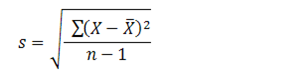S= sample standard deviation

Σ= sum of

n= number of scores in sample

Though this formula may appear to be a bit confusing, but you can break down and simplify it. You can understand step-by-step interactive process through example.

Step 1: Find out the mean

Step 2: Find out square of distance to mean for every data point

Step 3: Sum of the values gained from Step 2

Step 4: Divide it by number of data points

Step 5: Take up square root

While calculating standard deviation of sample you will identify that it comes with a slightly different formula:

s = √ [ Σ ( xi – x )2 / ( n – 1 ) ]

S is represented as sample standard deviation

X is sample mean

Xi is ith element from sample

n is number of elements found in sample

Standard deviation is equal to that of square root of variance.

While calculating the variance of any data set it is possible through taking arithmetic mean of squared difference between different value and mean value. Squaring different comes with three advantages:

1. Squaring makes each and every term very position so this means that value above mean would not have cancel values below mean
2. Squaring may add up more weight to larger differences and in some cases, there is an extra weight which is appropriate from mean that is highly significant
3. Mathematics may appear to be highly manageable while using this to measure subsequent statistical calculations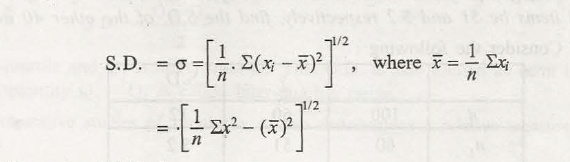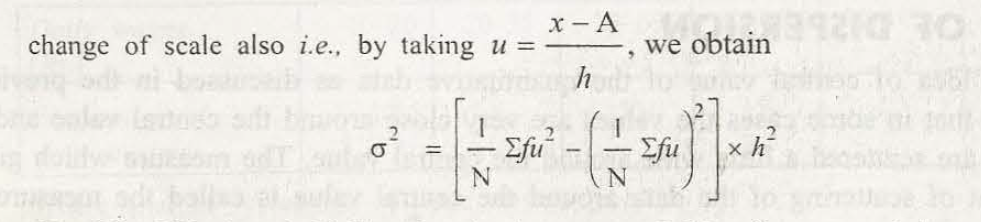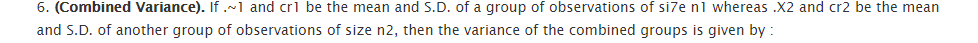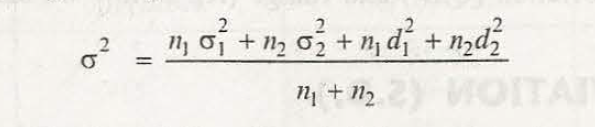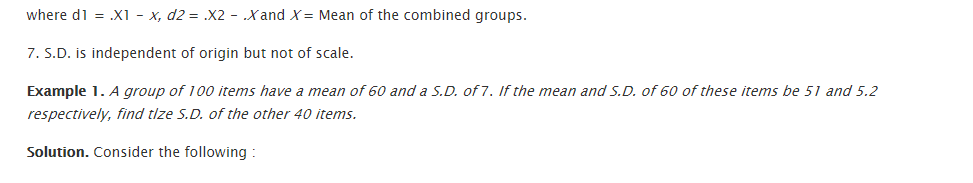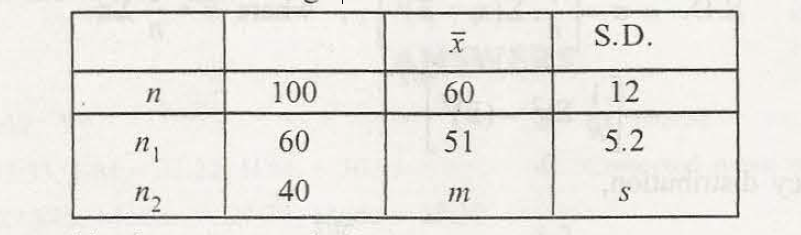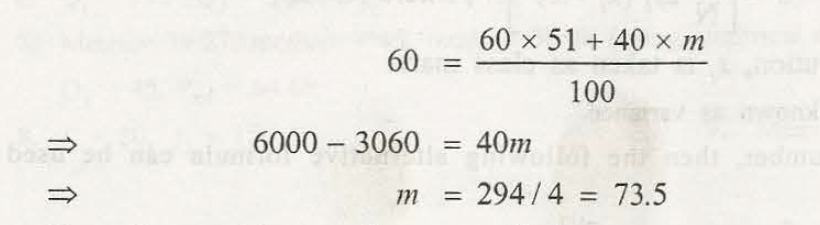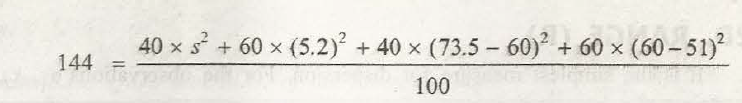### Customer Reviews

My Homework Help
Rated 5.0 out of 5 based on 510 customer reviews at